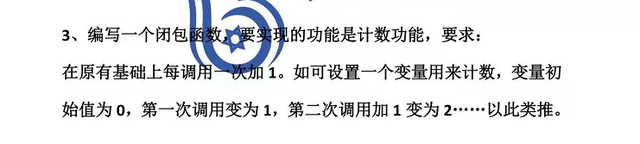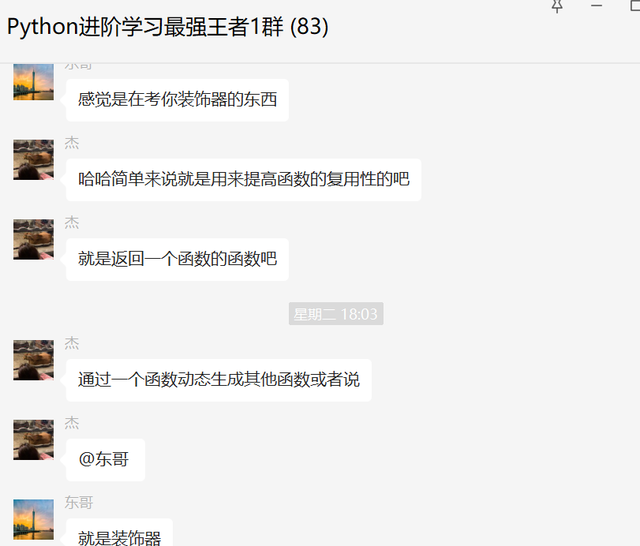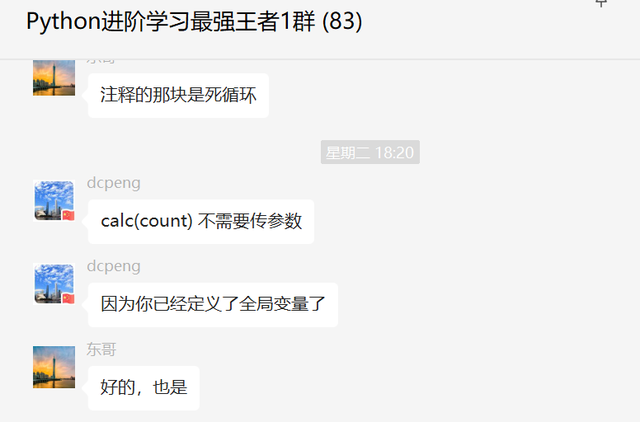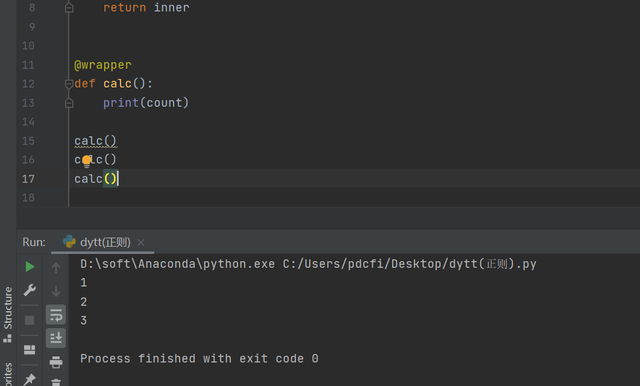# 一、前言# 二、解决过程``````count = 0
def wrapper(func):
def inner(*args, **kwargs):
global count
count += 1
result = func(*args, **kwargs)
return result
return inner

# while True:
#     @wrapper
#     def calc(count):
#         print(count)
#
#     calc(count)
for i in range(10):
@wrapper
def calc(count):
print(count)

calc(count)
````````````count = 0
def wrapper(func):
def inner(*args, **kwargs):
global count
count += 1
result = func(*args, **kwargs)
return result
return inner

@wrapper
def calc():
print(count)

calc()
``````